# Convergence (puctually and uniformly)

### Basic Concepts and Principles

We have seen the theory of Hilbert spaces there is a class of functions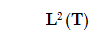for which the Fourier series is convergent. This convergence is in the sense of the norm generated by this space, called the quadratic norm, ie we have obtained.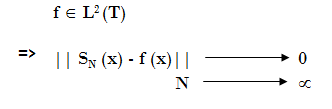Fourier series convergence to the midjump-value in jump-discontinuities points.

But it is important to note that this convergence is in the sense of the norm.

Moreover we have found that given a sequence {u} of elements in spaceThere exists a function of our space of functions for which {u} are the Fourier coefficients and of trigonometric series so formed, converges to the function in the norm sense of this spaceWe now want to extend a little more this space of functions and find conditions for puntual and uniform convergence of Fourier series.

It is a fact, in a finite measure space M, as a closed interval, it can possible to shown that if p < q then### Extended Theory

Specifically, for the definition of Fourier coefficients is necessary only makes sense that the function be in the space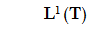because if

f ∈ L1(T) => ∫10 |f(x)| dx < ∞ then

||fn|| = |∫10f(x) e2πinx dx| ≤ ∫10|f(x)| |e2πinx |dx = ∫10|f(x)| dx = || f ||L1 < ∞

Thus the Fourier coefficients are well defined in this space

Lets consider now the normed space of functions E = (L1(T), || f ||L1)

|| f ||L1 = ∫10 |f(x)| dx

It is possible to show that E is Banach space (normed and complete).

Lets considert now consider the sequence space

l(Z) = {{an} : sup |an| < ∞}

We can define the normed space

H = (l(Z), || {an} ||l∞)

Where the norm is now

|| {an} ||l∞ = sup |an|

The space H so defined is also a Banach space.

We consider now the application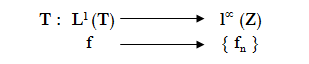In this case, it can be shown that the application T, is linear, continuous and injective but not surjective (as we had in the analog case of square integrable functions, see the section on Hilbert spaces).

To see that it is only necessary

Lemma (Riemann - Lebesgue)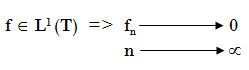From this it follows the assertion that T is not surjective, for example take the series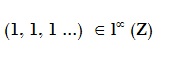Theorem of punctually Convergence 1 (Hölder's Criterion)
Let the function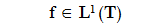Piecewise continuous, such that satisfies the Right-left Hölder condition then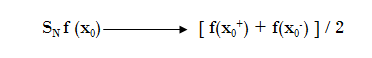The following is a theorem similar, except that the Dini condition is slightly weaker than Hölder condition
Theorem of punctually Convergence 1 (Dini's Criterion)
Let the functionand lets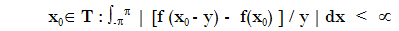then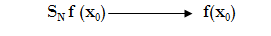Observation 1: differentiable functions verified trivially of Hölder' and Dini's Criterion because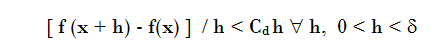Thus, f differentiable => Fourier series of f converges punctually

Observation 2: also this function satiesfies the Hölder' and Dini's Criterion although it is not differentiable in some pontis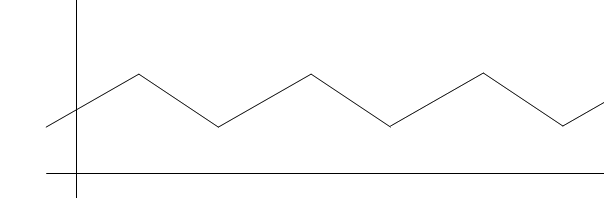Observation 3: There exist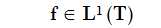such that its Fourier series diverges almost everywhere
Observation 4: Since 1968 we know that if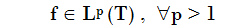Fourier series of f converges almost everywhere (p = 2 is a Carlesson's result, shown in 1966)

Theorem 1: Uniformly convergence of Fourier series
If we have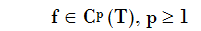Then the Fourier series converges uniformly and the convergence-ratio is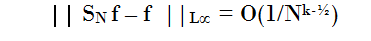Finally we will see a uniform convergence theorem in the context of Sobolev Spaces , This theorem provides us a intermediate function's space in which we can delete continuous or differentiable functions conditions, but to understand it, we see first the following lemma which returns the Fourier coefficients of the derivatives of a function (obviously, if it's differentiable) in terms of Fourier coefficients:

Lema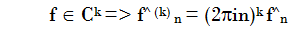Theorem 2: uniformly convergence of Fourier Series in the context of Sobolev spacesThen the Fourier series of f converges uniformly convergence with ratioWhat this important theorem says join with the previous Lemma is that the uniformly convergent of the Fourier series of a function is only necessary that the function has only a little more than "half derivative".
In summary, we have uniform convergence of Fourier Series of a function, it is only necessary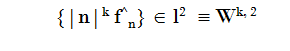# Was useful? want add anything?

Post here

### Post from other users

Post here